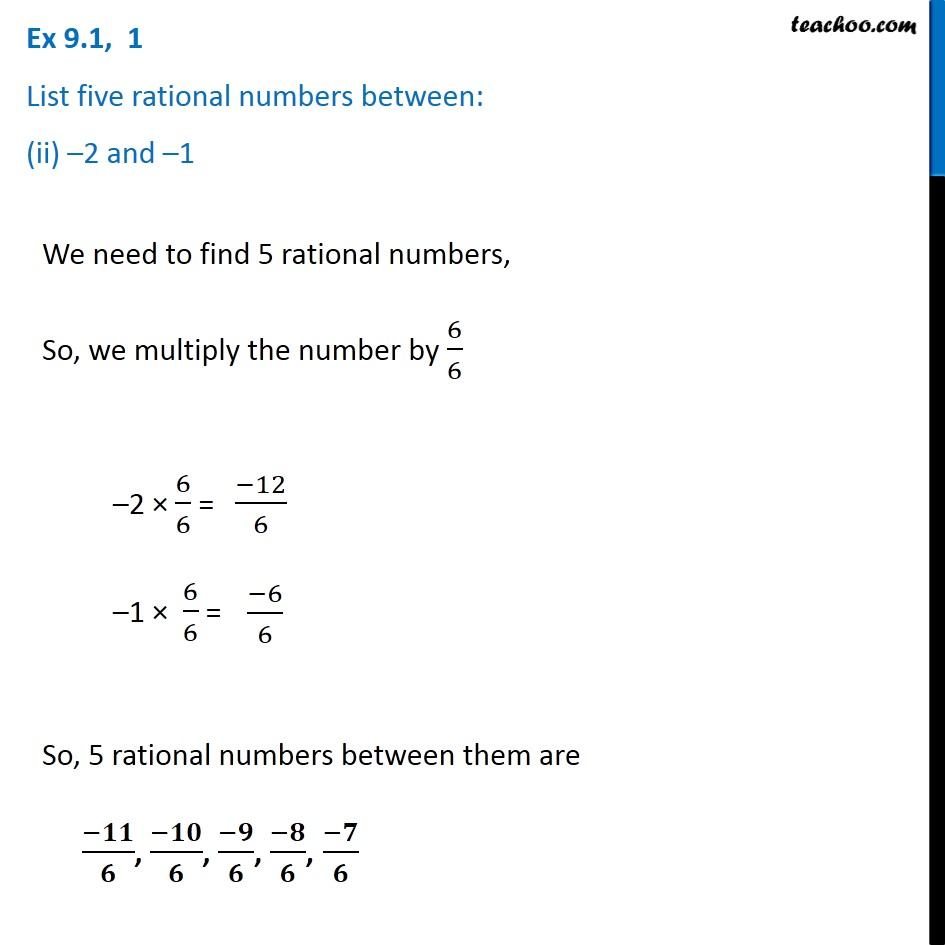Ex 9.1

Chapter 9 Class 7 Rational Numbers
Serial order wiseGet live Maths 1-on-1 Classs - Class 6 to 12

### Transcript

Ex 9.1, 1 List five rational numbers between: (ii) –2 and –1 We need to find 5 rational numbers, So, we multiply the number by 6/6 –2 × 6/6 = –1 × 6/6 = So, 5 rational numbers between them are (−𝟏𝟏)/𝟔, (−𝟏𝟎)/𝟔, (−𝟗)/𝟔, (−𝟖)/𝟔, (−𝟕)/𝟔